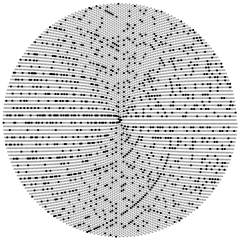# Check if a number is prime

yourbasic.org/golangSacks spiral of prime numbers

## Ints

For integer types, use `ProbablyPrime(0)` from package `math/big`. This primality test is 100% accurate for inputs less than 264.

``````const n = 1212121
if big.NewInt(n).ProbablyPrime(0) {
fmt.Println(n, "is prime")
} else {
fmt.Println(n, "is not prime")
}``````
``1212121 is prime``

## Larger numbers

For larger numbers, you need to provide the desired number of tests to ProbablyPrime(n). For n tests, the probability of returning true for a randomly chosen non-prime is at most (1/4)n. A common choice is to use n = 20; this gives a false positive rate 0.000,000,000,001.

``````z := new(big.Int)
fmt.Sscan("170141183460469231731687303715884105727", z)
if z.ProbablyPrime(20) {
fmt.Println(z, "is probably prime")
} else {
fmt.Println(z, "is not prime")
}``````
``170141183460469231731687303715884105727 is probably prime``

### More code examplesGo blueprints: code for com­mon tasks is a collection of handy code examples.